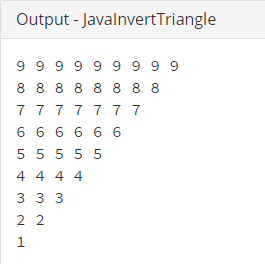## Java Programming Examples Tutorial Index

This Java program is used to print Invert Triangle.

Example:

``````public class JavaInvertTriangle
{
public static void main(String args[])
{
int num = 9;
while(num > 0)
{
for(int i=1; i<=num; i++)
{
System.out.print(" "+num+" ");
}
System.out.print("\n");
num--;
}
}
}
``````

Program Output:Explanation:

This program creates a design of an inverse triangle in the form of numbers. In this program first you need to create a class name 'JavaInvertTriangle' and the main() method within the class. Now inside the main() definition, declare an integer type variable 'num' and assign it with a value 9.Now you need to imply a loop statement to repeat the numbers from 9 up to 1 to create the design of an inverse triangle something like this:

Now the loop will continue if the num is having value greater than 0. Within the while loop, comes the for loop. The statement:

``for(int i=1; i<=num; i++)``

counts the integer variable i from 1 till the value of 'num' (here from 1 to 9) and within the scope of the for - loop, the value of 'i' is getting printed. As the curly braces of the for - loop ends, the printing cursor goes to the next line using the escape sequence '\n' (i.e. new line) and the value of num decreases by 1, using the post decrement operator. The decrement of 'num' value goes till one and hence prints the triangle from upper to lower starting from 9 nine times till 1, a single time.